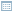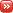• ## One Over Square Root of N

Posted by Konstantin 04.12.2011

There is one rule of thumb that I find quite useful and happen to use fairly often. It is probably not widely known nor described in textbooks (I stumbled upon it on my own), so I regularly have to explain it.  Next time I'll just point out to this post.

The rule is the following: a proportion estimate obtained on a sample of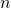points should only be trusted up to an error of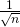.

For example, suppose that you read in the newspaper that "25% of students like statistics". Now, if this result has been obtained from a survey of 64 participants, you should actually interpret the answer as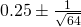, that is,, which means that the actual percentage lies somewhere between 12.5% and 37.5%.

As another example, in machine learning, you often get to see cases where someone evaluates two classification algorithms on a test set of, say, 400 instances, measures that the first algorithm has a precision of 90%, the second a precision of, say, 92%, and boldly claims the dominance of the second algorithm. At this point, without going deeply into statistics, it is easy to figure that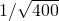should be somewhere around 5%, hence the difference between 90% and 92% is not too significant to celebrate.

### The Explanation

The derivation of the rule is fairly straightforward. Consider a Bernoulli-distributed random variable with parameter. We then take an i.i.d. sample of size, and use it to estimate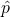:The 95% confidence interval for this estimate, computed using the normal approximation is then: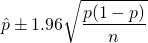What remains is to note thatand that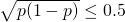. By substituting those two approximations we immediately get that the interval is at most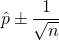### Limitations

It is important to understand the limitations of the rule. In the cases where the true proportion estimate isandis large enough for the normal approximation to make sense (20 is already good), the one-over-square-root-of-n rule is very close to a true 95% confidence interval.

When the true proportion estimate is closer to 0 or 1, however,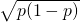is not close to 0.5 anymore, and the rule of thumb results in a conservatively large interval.

In particular, the true 95% confidence interval for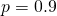will be nearly two times smaller (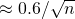). For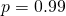the actual interval is five times smaller (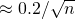). However, given the simplicity of the rule, the fact that the trueis rarely so close to 1, and the idea that it never hurts to be slightly conservative in statistical estimates, I'd say the one-over-a-square-root-of-n rule is a practically useful tool in most situations.

Posted by Konstantin @ 2:51 am

• ### 1 Comment

1. Ankur on 11.01.2012 at 15:53 (Reply)

Hallo Konstantin,

thanks for the post. I remember learning this during my masters but couldnt remember what this thumb rule wass called or why it is so.. so I finally searched for 'one over root n'. Apparently Google only works if you know what you're looking for 🙂

I think it's a super helpful tool because of its simplicity! (Not easy to explain to laymen or people in the business world why the 1/root(n) but good for a confidence estimation for oneself!)

Best
Ankur

Please note: Comment moderation is enabled and may delay your comment. There is no need to resubmit your comment.

### Calendar

December 2011
M T W T F S S
« Oct   Jan »
1234
567891011
12131415161718
19202122232425
262728293031